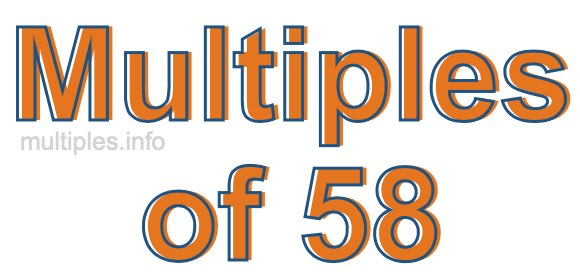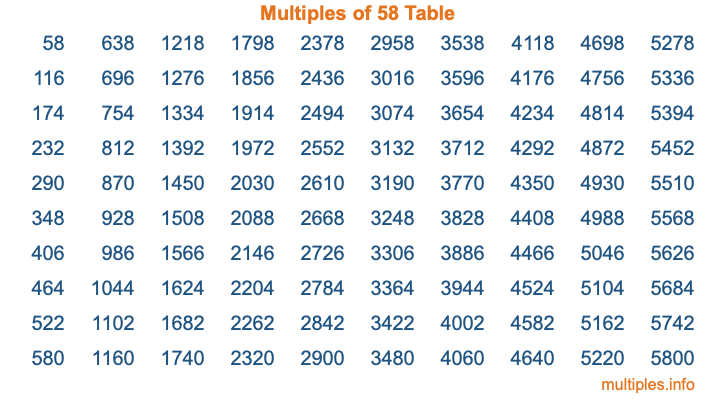Multiples of 58Welcome to the Multiples of 58 page. Here we will first teach you everything you will ever need to know about the multiples of 58, and then give you a study guide summary of everything we taught you to make sure you remember it all. Use this page to look up facts and learn information about the multiples of 58. This page will make you a multiples of fifty-eight expert!

Definition of Multiples of 58
Multiples of 58 are all the numbers that when divided by 58 equal an integer. Each of the multiples of 58 are called a multiple. A multiple of 58 is created by multiplying 58 by an integer.

Therefore, to create a list of multiples of 58, you start with 1 multiplied by 58, then 2 multiplied by 58, then 3 multiplied by 58, and so on for as long as you want. Thus, the list of the first five multiples of 58 is 58, 116, 174, 232, and 290. To see a larger list of multiples of 58, see the printable image of Multiples of 58 further down on this page. We also have a category where you can choose any nth multiple of 58.

Multiples of 58 Checker
The Multiples of 58 Checker below checks to see if any number of your choice is a multiple of 58. In other words, it checks to see if there is any number (integer) that when multiplied by 58 will equal your number. To do that, we divide your number by 58. If the the quotient is an integer, then your number is a multiple of 58.

Is  a multiple of 58?

Least Common Multiple of 58 and ...
A Least Common Multiple (LCM) is the lowest multiple that two or more numbers have in common. This is also called the smallest common multiple or lowest common multiple and is useful to know when you are adding our subtracting fractions. Enter one or more numbers below (58 is already entered) to find the LCM.

Check out our LCM Calculator if you need more details about the Least Common Multiple or if you need the LCM for different numbers for adding and subtraction fractions.

nth Multiple of 58
As we stated above, 58 is the first multiple of 58, 116 is the second multiple of 58, 174 is the third multiple of 58, and so on. Enter a number below to find the nth multiple of 58.

th multiple of 58

Multiples of 58 vs Factors of 58
58 is a multiple of 58 and a factor of 58, but that is where the similarities end. All postive multiples of 58 are 58 or greater than 58. All positive factors of 58 are 58 or less than 58.

Below is the beginning list of multiples of 58 and the factors of 58 so you can compare:

Multiples of 58: 58, 116, 174, 232, 290, etc.

Factors of 58: 1, 2, 29, 58

As you can see, the multiples of 58 are all the numbers that you can divide by 58 to get a whole number. The factors of 58, on the other hand, are all the whole numbers that you can multiply by another whole number to get 58.

It's also interesting to note that if a number (x) is a factor of 58, then 58 will also be a multiple of that number (x).

Multiples of 58 vs Divisors of 58
The divisors of 58 are all the integers that 58 can be divided by evenly. Below is a list of the divisors of 58.

Divisors of 58: 1, 2, 29, 58

The interesting thing to note here is that if you take any multiple of 58 and divide it by a divisor of 58, you will see that the quotient is an integer.

Multiples of 58 Table
Below is an image of the first 100 multiples of 58 in a table. The table is in chronological order, column by column. The first column has the first ten multiples of 58, the second column has the next ten multiples of 58, and so on.The Multiples of 58 Table is also referred to as the 58 Times Table or Times Table of 58. You are welcome to print out our table for your studies.

Negative Multiples of 58
Although not often discussed or needed in math, it is worth mentioning that you can make a list of negative multiples of 58 by multiplying 58 by -1, then by -2, then by -3, and so on, to get the following list of negative multiples of 58:

-58, -116, -174, -232, -290, etc.

Multiples of 58 Summary
Below is a summary of important Multiples of 58 facts that we have discussed on this page. To retain the knowledge on this page, we recommend that you read through the summary and explain to yourself or a study partner why they hold true.

There are an infinite number of multiples of 58.

A multiple of 58 divided by 58 will equal a whole number.

58 divided by a factor of 58 equals a divisor of 58.

The nth multiple of 58 is n times 58.

The largest factor of 58 is equal to the first positive multiple of 58.

58 is a multiple of every factor of 58.

58 is a multiple of 58.

A multiple of 58 divided by a divisor of 58 equals an integer.

58 divided by a divisor of 58 equals a factor of 58.

Any integer times 58 will equal a multiple of 58.

Multiples of a Number
Here you can get the multiples of another number, all with the same attention to detail as we did for multiples of 58 on this page.

Multiples of
Multiples of 59
Did you find our page about multiples of fifty-eight educational? Do you want more knowledge? Check out the multiples of the next number on our list!